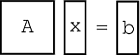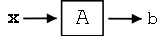# 14.1 Eigenvectors and eigenvalues

 Page 1 / 1
This module defines eigenvalues and eigenvectors and explains a method of finding them given a matrix. These ideas are presented, along with many examples, in hopes of leading up to an understanding of the Fourier Series.

In this section, our linear systems will be n×n matrices of complex numbers. For a little background into some of theconcepts that this module is based on, refer to the basics of linear algebra .

## Eigenvectors and eigenvalues

Let $A$ be an n×n matrix, where $A$ is a linear operator on vectors in $\mathbb{C}^{n}$ .

$Ax=b$
where $x$ and $b$ are n×1 vectors ( [link] ).Illustration of linear system and vectors.
eigenvector
An eigenvector of $A$ is a vector $v\in \mathbb{C}^{n}$ such that
$Av=\lambda v$
where $\lambda$ is called the corresponding eigenvalue . $A$ only changes the length of $v$ , not its direction.

## Graphical model

Through [link] and [link] , let us look at the difference between [link] and [link] .

If $v$ is an eigenvector of $A$ , then only its length changes. See [link] and notice how our vector's length is simply scaled by our variable, $\lambda$ , called the eigenvalue :

When dealing with a matrix $A$ , eigenvectors are the simplest possible vectors to operate on.

## Examples

From inspection and understanding of eigenvectors, find the two eigenvectors, ${v}_{1}$ and ${v}_{2}$ , of $A=\begin{pmatrix}3 & 0\\ 0 & -1\\ \end{pmatrix}()$ Also, what are the corresponding eigenvalues, ${\lambda }_{1}$ and ${\lambda }_{2}$ ? Do not worry if you are having problems seeing these values from the information given so far,we will look at more rigorous ways to find these values soon.

The eigenvectors you found should be: ${v}_{1}=\left(\begin{array}{c}1\\ 0\end{array}\right)$ ${v}_{2}=\left(\begin{array}{c}0\\ 1\end{array}\right)$ And the corresponding eigenvalues are ${\lambda }_{1}=3$ ${\lambda }_{2}=-1$

Show that these two vectors, ${v}_{1}=\left(\begin{array}{c}1\\ 1\end{array}\right)$ ${v}_{2}=\left(\begin{array}{c}1\\ -1\end{array}\right)$ are eigenvectors of $A$ , where $A=\begin{pmatrix}3 & -1\\ -1 & 3\\ \end{pmatrix}$ . Also, find the corresponding eigenvalues.

In order to prove that these two vectors are eigenvectors, we will show that these statements meetthe requirements stated in the definition . $A{v}_{1}=\begin{pmatrix}3 & -1\\ -1 & 3\\ \end{pmatrix}()\left(\begin{array}{c}1\\ 1\end{array}\right)()=\left(\begin{array}{c}2\\ 2\end{array}\right)()$ $A{v}_{2}=\begin{pmatrix}3 & -1\\ -1 & 3\\ \end{pmatrix}()\left(\begin{array}{c}1\\ -1\end{array}\right)()=\left(\begin{array}{c}4\\ -4\end{array}\right)()$ These results show us that $A$ only scales the two vectors ( i.e. changes their length) and thus it proves that [link] holds true for the following two eigenvalues that you were asked to find: ${\lambda }_{1}=2$ ${\lambda }_{2}=4$ If you need more convincing, then one could also easilygraph the vectors and their corresponding product with $A$ to see that the results are merely scaled versions of our original vectors, ${v}_{1}$ and ${v}_{2}$ .

## Calculating eigenvalues and eigenvectors

In the above examples, we relied on your understanding of thedefinition and on some basic observations to find and prove the values of the eigenvectors and eigenvalues. However, as youcan probably tell, finding these values will not always be that easy. Below, we walk through a rigorous and mathematicalapproach at calculating the eigenvalues and eigenvectors of a matrix.

## Finding eigenvalues

Find $\lambda \in \mathbb{C}$ such that $v\neq 0$ , where $0$ is the "zero vector." We will start with [link] , and then work our way down until we find a way to explicitly calculate $\lambda$ . $Av=\lambda v$ $Av-\lambda v=0$ $(A-\lambda I)v=0$ In the previous step, we used the fact that $\lambda v=\lambda Iv$ where $I$ is the identity matrix. $I=\begin{pmatrix}1 & 0 & \dots & 0\\ 0 & 1 & \dots & 0\\ 0 & 0 & \ddots & ⋮\\ 0 & \dots & \dots & 1\\ \end{pmatrix}()$ So, $A-\lambda I$ is just a new matrix.

Given the following matrix, $A$ , then we can find our new matrix, $A-\lambda I$ . $A=\begin{pmatrix}a_{1, 1} & a_{1, 2}\\ a_{2, 1} & a_{2, 2}\\ \end{pmatrix}()$ $A-\lambda I=\begin{pmatrix}a_{1, 1}-\lambda & a_{1, 2}\\ a_{2, 1} & a_{2, 2}-\lambda \\ \end{pmatrix}()$

If $(A-\lambda I)v=0$ for some $v\neq 0$ , then $A-\lambda I$ is not invertible . This means: $\det (A-\lambda I)=0$ This determinant (shown directly above) turns out to be a polynomial expression (of order $n$ ). Look at the examples below to see what this means.

Starting with matrix $A$ (shown below), we will find the polynomial expression, where our eigenvalues will be the dependent variable. $A=\begin{pmatrix}3 & -1\\ -1 & 3\\ \end{pmatrix}()$ $A-\lambda I=\begin{pmatrix}3-\lambda & -1\\ -1 & 3-\lambda \\ \end{pmatrix}()$ $\det (A-\lambda I)=(3-\lambda )^{2}--1()^{2}=\lambda ^{2}-6\lambda +8$ $\lambda =\{2, 4\}()$

Starting with matrix $A$ (shown below), we will find the polynomial expression, where our eigenvalues will be the dependent variable. $A=\begin{pmatrix}a_{1, 1} & a_{1, 2}\\ a_{2, 1} & a_{2, 2}\\ \end{pmatrix}()$ $A-\lambda I=\begin{pmatrix}a_{1, 1}-\lambda & a_{1, 2}\\ a_{2, 1} & a_{2, 2}-\lambda \\ \end{pmatrix}()$ $\det (A-\lambda I)=\lambda ^{2}-(a_{1, 1}+a_{2, 2})\lambda -a_{2, 1}a_{1, 2}+a_{1, 1}a_{2, 2}$

If you have not already noticed it, calculating the eigenvalues is equivalent to calculating the roots of $\det (A-\lambda I)={c}_{n}\lambda ^{n}+{c}_{n-1}\lambda ^{(n-1)}+\dots +{c}_{1}\lambda +{c}_{0}=0$

Therefore, by simply using calculus to solve for the roots of our polynomial we can easily find the eigenvalues of ourmatrix.

## Finding eigenvectors

Given an eigenvalue, ${\lambda }_{i}$ , the associated eigenvectors are given by $Av={\lambda }_{i}v$ $A\left(\begin{array}{c}{v}_{1}\\ ⋮\\ {v}_{n}\end{array}\right)=\left(\begin{array}{c}{\lambda }_{1}{v}_{1}\\ ⋮\\ {\lambda }_{n}{v}_{n}\end{array}\right)$ set of $n$ equations with $n$ unknowns. Simply solve the $n$ equations to find the eigenvectors.

## Main point

Say the eigenvectors of $A$ , $\{{v}_{1}, {v}_{2}, \dots , {v}_{n}\}()$ , span $\mathbb{C}^{n}$ , meaning $\{{v}_{1}, {v}_{2}, \dots , {v}_{n}\}()$ are linearly independent and we can write any $x\in \mathbb{C}^{n}$ as

$x={\alpha }_{1}{v}_{1}+{\alpha }_{2}{v}_{2}+\dots +{\alpha }_{n}{v}_{n}$
where $\{{\alpha }_{1}, {\alpha }_{2}, \dots , {\alpha }_{n}\}()\in \mathbb{C}$ . All that we are doing is rewriting $x$ in terms of eigenvectors of $A$ . Then, $Ax=A({\alpha }_{1}{v}_{1}+{\alpha }_{2}{v}_{2}+\dots +{\alpha }_{n}{v}_{n})$ $Ax={\alpha }_{1}A{v}_{1}+{\alpha }_{2}A{v}_{2}+\dots +{\alpha }_{n}A{v}_{n}$ $Ax={\alpha }_{1}{\lambda }_{1}{v}_{1}+{\alpha }_{2}{\lambda }_{2}{v}_{2}+\dots +{\alpha }_{n}{\lambda }_{n}{v}_{n}=b$ Therefore we can write, $x=\sum {\alpha }_{i}{v}_{i}$ and this leads us to the following depicted system:Depiction of system where we break our vector, x , into a sum of its eigenvectors.

where in [link] we have, $b=\sum {\alpha }_{i}{\lambda }_{i}{v}_{i}$

By breaking up a vector, $x$ , into a combination of eigenvectors, the calculation of $Ax$ is broken into "easy to swallow" pieces.

## Practice problem

For the following matrix, $A$ and vector, $x$ , solve for their product. Try solving it using two differentmethods: directly and using eigenvectors. $A=\begin{pmatrix}3 & -1\\ -1 & 3\\ \end{pmatrix}()$ $x=\left(\begin{array}{c}5\\ 3\end{array}\right)()$

Direct Method (use basic matrix multiplication) $Ax=\begin{pmatrix}3 & -1\\ -1 & 3\\ \end{pmatrix}()\left(\begin{array}{c}5\\ 3\end{array}\right)()=\left(\begin{array}{c}12\\ 4\end{array}\right)()$ Eigenvectors (use the eigenvectors and eigenvalues we found earlier for this same matrix) ${v}_{1}=\left(\begin{array}{c}1\\ 1\end{array}\right)$ ${v}_{2}=\left(\begin{array}{c}1\\ -1\end{array}\right)$ ${\lambda }_{1}=2$ ${\lambda }_{2}=4$ As shown in [link] , we want to represent $x$ as a sum of its scaled eigenvectors. For this case, we have: $x=4{v}_{1}+{v}_{2}$ $x=\left(\begin{array}{c}5\\ 3\end{array}\right)()=4\left(\begin{array}{c}1\\ 1\end{array}\right)()+\left(\begin{array}{c}1\\ -1\end{array}\right)()$ $Ax=A(4{v}_{1}+{v}_{2})={\lambda }_{i}(4{v}_{1}+{v}_{2})$ Therefore, we have $Ax=4\times 2\left(\begin{array}{c}1\\ 1\end{array}\right)()+4\left(\begin{array}{c}1\\ -1\end{array}\right)()=\left(\begin{array}{c}12\\ 4\end{array}\right)()$ Notice that this method using eigenvectors required no matrix multiplication. This may have seemed more complicated here, but just imagine $A$ being really big, or even just a few dimensions larger!

#### Questions & Answers

Is there any normative that regulates the use of silver nanoparticles?
what king of growth are you checking .?
Renato
What fields keep nano created devices from performing or assimulating ? Magnetic fields ? Are do they assimilate ?
why we need to study biomolecules, molecular biology in nanotechnology?
?
Kyle
yes I'm doing my masters in nanotechnology, we are being studying all these domains as well..
why?
what school?
Kyle
biomolecules are e building blocks of every organics and inorganic materials.
Joe
anyone know any internet site where one can find nanotechnology papers?
research.net
kanaga
sciencedirect big data base
Ernesto
Introduction about quantum dots in nanotechnology
what does nano mean?
nano basically means 10^(-9). nanometer is a unit to measure length.
Bharti
do you think it's worthwhile in the long term to study the effects and possibilities of nanotechnology on viral treatment?
absolutely yes
Daniel
how to know photocatalytic properties of tio2 nanoparticles...what to do now
it is a goid question and i want to know the answer as well
Maciej
characteristics of micro business
Abigail
for teaching engĺish at school how nano technology help us
Anassong
Do somebody tell me a best nano engineering book for beginners?
there is no specific books for beginners but there is book called principle of nanotechnology
NANO
what is fullerene does it is used to make bukky balls
are you nano engineer ?
s.
fullerene is a bucky ball aka Carbon 60 molecule. It was name by the architect Fuller. He design the geodesic dome. it resembles a soccer ball.
Tarell
what is the actual application of fullerenes nowadays?
Damian
That is a great question Damian. best way to answer that question is to Google it. there are hundreds of applications for buck minister fullerenes, from medical to aerospace. you can also find plenty of research papers that will give you great detail on the potential applications of fullerenes.
Tarell
what is the Synthesis, properties,and applications of carbon nano chemistry
Mostly, they use nano carbon for electronics and for materials to be strengthened.
Virgil
is Bucky paper clear?
CYNTHIA
carbon nanotubes has various application in fuel cells membrane, current research on cancer drug,and in electronics MEMS and NEMS etc
NANO
so some one know about replacing silicon atom with phosphorous in semiconductors device?
Yeah, it is a pain to say the least. You basically have to heat the substarte up to around 1000 degrees celcius then pass phosphene gas over top of it, which is explosive and toxic by the way, under very low pressure.
Harper
Do you know which machine is used to that process?
s.
how to fabricate graphene ink ?
for screen printed electrodes ?
SUYASH
What is lattice structure?
of graphene you mean?
Ebrahim
or in general
Ebrahim
in general
s.
Graphene has a hexagonal structure
tahir
On having this app for quite a bit time, Haven't realised there's a chat room in it.
Cied
what is biological synthesis of nanoparticles
Got questions? Join the online conversation and get instant answers!By Jordon HumphreysBy Sam LuongBy Nick Swain